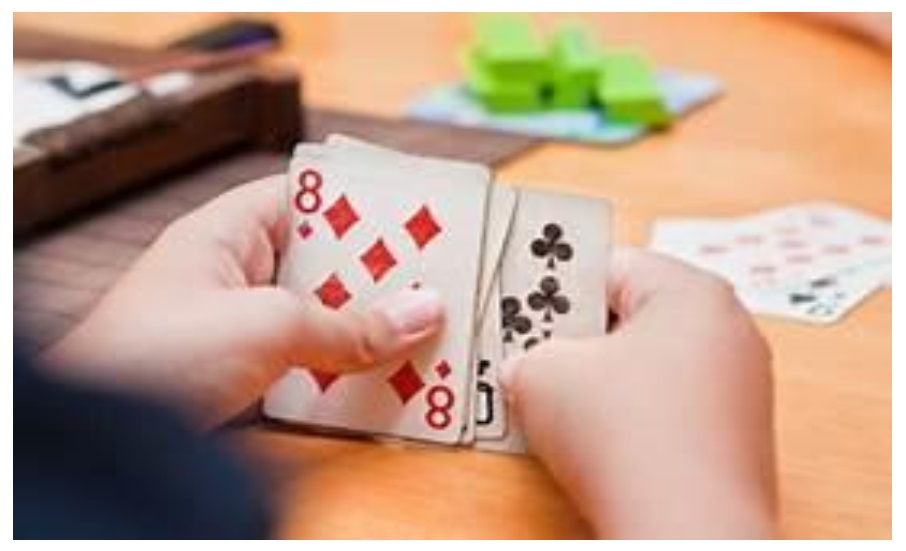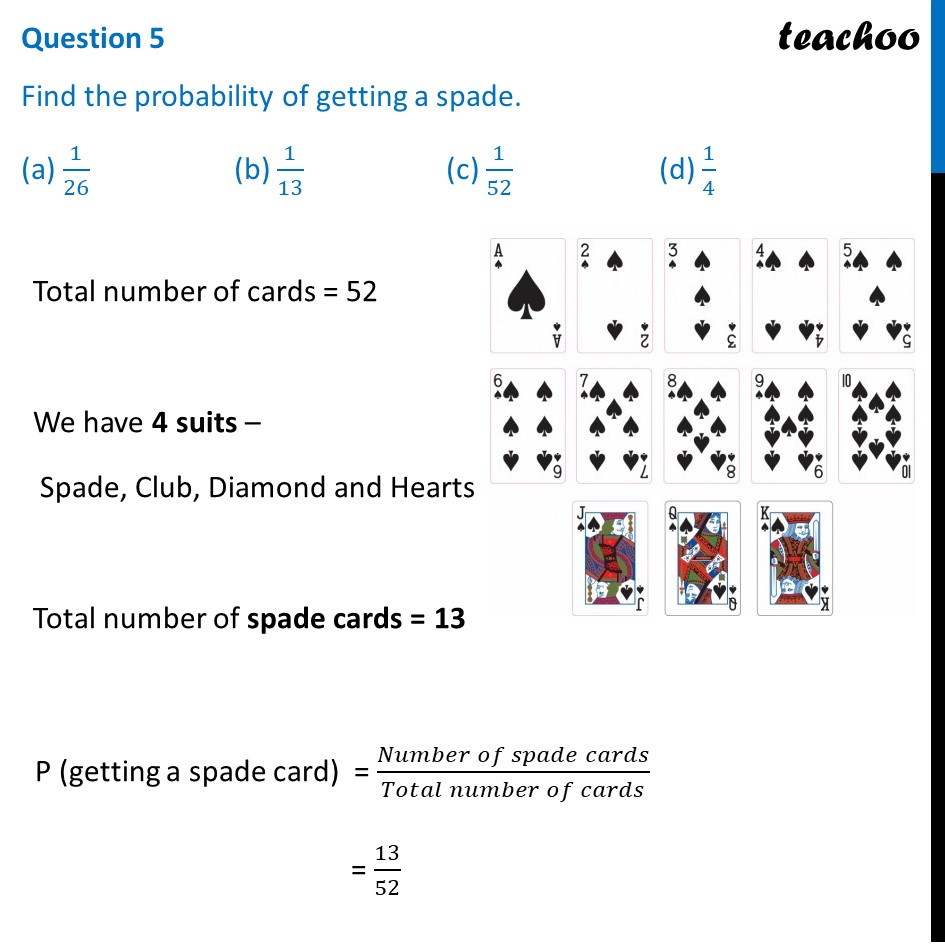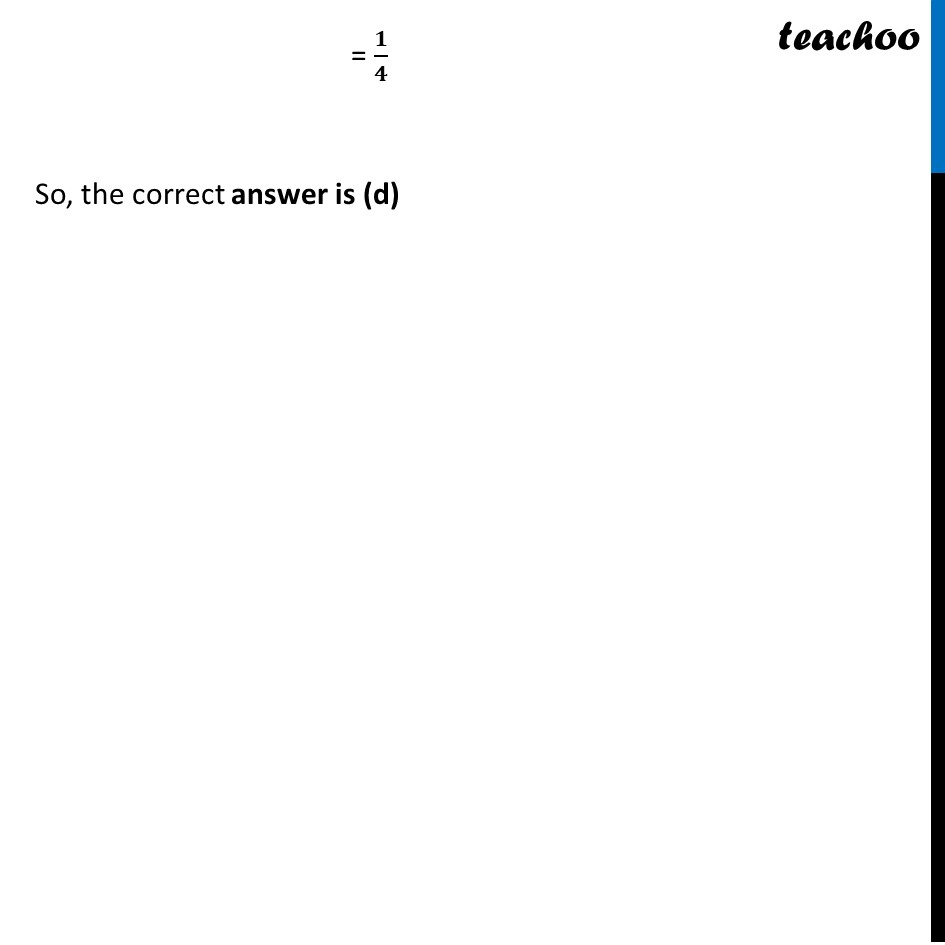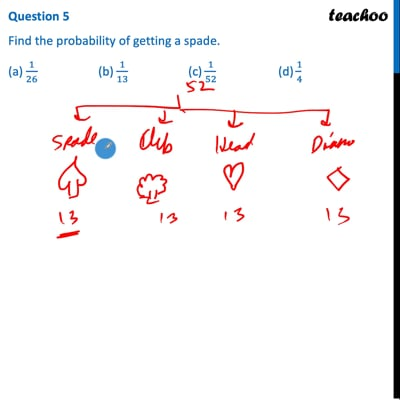Case Based Questions (MCQ)

Chapter 15 Class 10 Probability
Serial order wise
This question is inspired from Ex 15.1, 14 - Chapter 15 Class 10 - Probability

## On a weekend Rani was playing cards with her family .The deck has 52 cards. If her brother drew one card .##This video is only available for Teachoo black users

Maths Crash Course - Live lectures + all videos + Real time Doubt solving!

### Transcript

Question On a weekend Rani was playing cards with her family .The deck has 52 cards. If her brother drew one card .Question 1 Find the probability of getting a king of red colour (a) 1/26 (b) 1/13 (c) 1/52 (d) 1/4Total number of cards = 52 Total number of kings of red colour = 2 P (getting a king of red colour) = (𝑁𝑢𝑚𝑏𝑒𝑟 𝑜𝑓 𝑘𝑖𝑛𝑔 𝑐𝑎𝑟𝑑𝑠 𝑜𝑓 𝑟𝑒𝑑 𝑐𝑜𝑙𝑜𝑢𝑟)/(𝑇𝑜𝑡𝑎𝑙 𝑛𝑢𝑚𝑏𝑒𝑟 𝑜𝑓 𝑐𝑎𝑟𝑑𝑠) = 2/52 = 𝟏/𝟐𝟔 So, the correct answer is (a) Question 2 Find the probability of getting a face card. (a) 1/26 (b) 1/13 (c) 2/13 (d) 3/13Total number of cards = 52 Face cards are King, Queen and Jack Each type has 4 cards Total number of face cards = 3 × 4 = 12 P (getting a face card) = (𝑁𝑢𝑚𝑏𝑒𝑟 𝑜𝑓 𝑓𝑎𝑐𝑒 𝑐𝑎𝑟𝑑𝑠)/(𝑇𝑜𝑡𝑎𝑙 𝑛𝑢𝑚𝑏𝑒𝑟 𝑜𝑓 𝑐𝑎𝑟𝑑𝑠) = 12/52 = 𝟑/𝟏𝟑 So, the correct answer is (d) Question 3 Find the probability of getting a jack of hearts (a) 1/26 (b) 1/52 (c) 3/52 (d) 3/26Total number of cards = 52 We have 4 Jack cards Total number of Jack of hearts = 1 P (getting a Jack of hearts) = (𝑁𝑢𝑚𝑏𝑒𝑟 𝑜𝑓 𝐽𝑎𝑐𝑘 𝑜𝑓 ℎ𝑒𝑎𝑟𝑡𝑠 𝑐𝑎𝑟𝑑𝑠)/(𝑇𝑜𝑡𝑎𝑙 𝑛𝑢𝑚𝑏𝑒𝑟 𝑜𝑓 𝑐𝑎𝑟𝑑𝑠) = 𝟏/𝟓𝟐 So, the correct answer is (b) Question 4 Find the probability of getting a red face card (a) 3/26 (b) 1/13 (c) 1/52 (d) 1/4 Total number of cards = 52 Face cards are King, Queen and Jack Total number Red face cards = 6 P (getting a red face card) = (𝑁𝑢𝑚𝑏𝑒𝑟 𝑜𝑓 𝑟𝑒𝑑 𝑓𝑎𝑐𝑒 𝑐𝑎𝑟𝑑𝑠)/(𝑇𝑜𝑡𝑎𝑙 𝑛𝑢𝑚𝑏𝑒𝑟 𝑜𝑓 𝑐𝑎𝑟𝑑𝑠) = 6/52 = 𝟑/𝟐𝟔 So, the correct answer is (a) Question 5 Find the probability of getting a spade. (a) 1/26 (b) 1/13 (c) 1/52 (d) 1/4Total number of cards = 52 We have 4 suits – Spade, Club, Diamond and Hearts Total number of spade cards = 13 P (getting a spade card) = (𝑁𝑢𝑚𝑏𝑒𝑟 𝑜𝑓 𝑠𝑝𝑎𝑑𝑒 𝑐𝑎𝑟𝑑𝑠)/(𝑇𝑜𝑡𝑎𝑙 𝑛𝑢𝑚𝑏𝑒𝑟 𝑜𝑓 𝑐𝑎𝑟𝑑𝑠) = 13/52 = 𝟏/𝟒 So, the correct answer is (d)